# Thom isomorphism

An isomorphism between the (generalized) (co)homology groups of the base space of a vector (sphere) bundleand the (co)homology groups of its Thom space.
Suppose the-dimensional vector bundleover a finite cell complexis oriented in some multiplicative generalized cohomology theory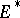(cf. Generalized cohomology theories), that is, there exists a Thom class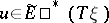. Then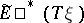is an-module, and the homomorphism, given by multiplication by the Thom class, is an isomorphism, called the Thom isomorphism (or Thom–Dold isomorphism).
There is a dually-defined isomorphism.
In the case whereis the classical cohomology theory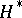, the isomorphism is described in , and it was established for an arbitrary theoryin . Moreover, ifis not oriented in the integral cohomology theory, then there is an isomorphism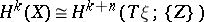, where the right-hand side is the cohomology group with coefficients in the local system of groups. More generally, ifis non-oriented in the cohomology theory, there is an isomorphism which generalizes both the Thom isomorphism described above and the Thom–Dold isomorphism for-oriented bundles .